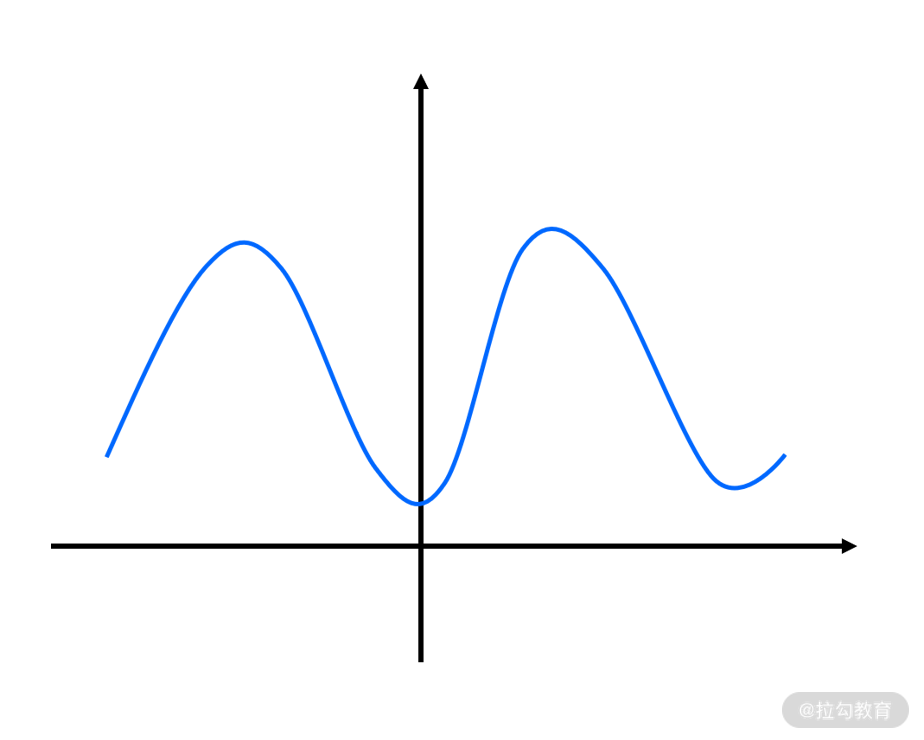05 求极值：如何找到复杂业务的最优解？

形式化定义

“形式化定义”，就是用函数去表达需要用文字描述的问题。也就是说，在做决策之前，需要把你的动作、收益、风险，用函数建立起联系。

r(x) = p(x)×(m - x - c)

追逐收益最大化

“追逐收益最大化”就是求解这个函数的最值，可能是最大值、也可能是最小值。

【例题1】假设你是某电商公司营销系统的工程师。你们某个商品的购买概率和补贴额的关系为，p(x) = 0.05 x + 0.2。该商品原价 m 为 16 元，成本价 c 为 8 元，求利润最大的补贴额应该是多少？

【解析】商品的利润函数为 r(x) = p(x)×(m - x - c) = (0.05x + 0.2)×(16 - x - 8) = -0.05x2 + 0.2x + 1.6，利用高中的数学求导法，令 r(x) 的导函数为零并解方程，则有：

r'(x) = -0.1x+0.2 = 0

def getSubsidy(k,b,m,c):

rx = [-k, k*(m-c)-b, b*(m-c)]

rpx = [-2*k, k*(m-c)-b]

return -rpx/rpx

方程解不出来怎么办？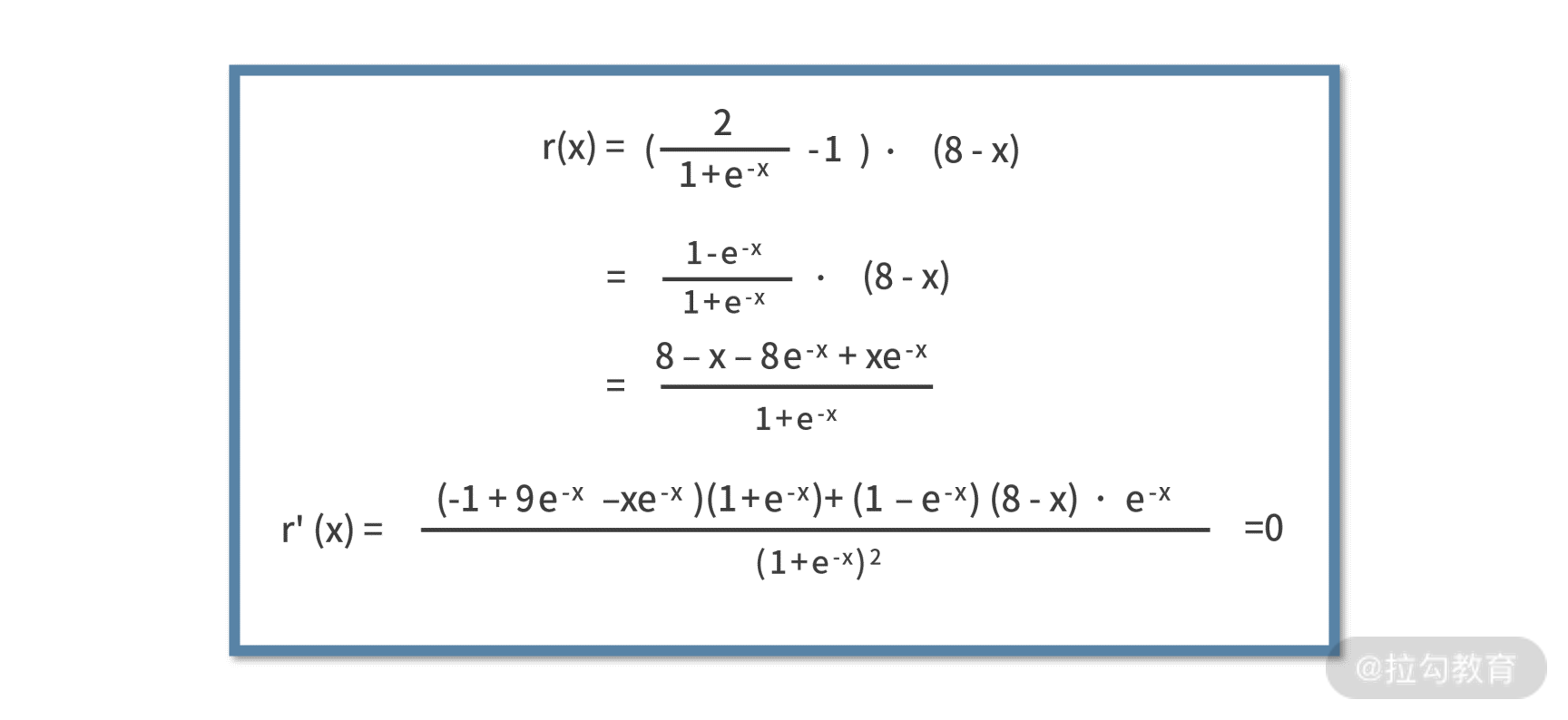梯度下降法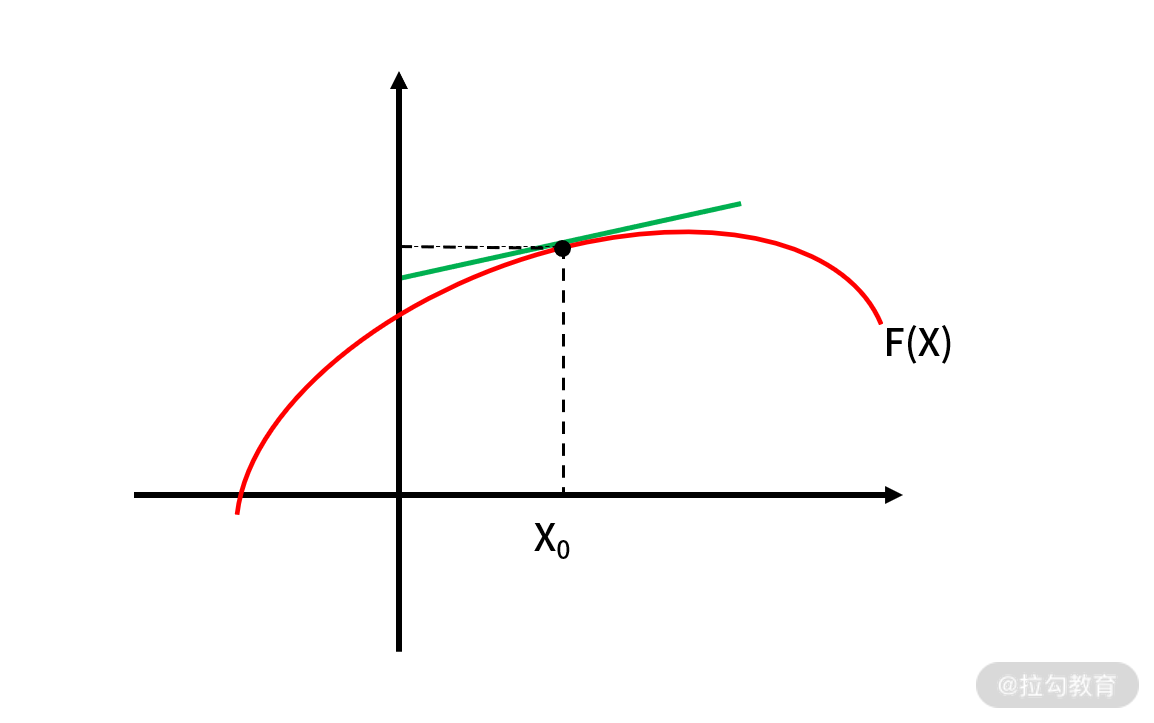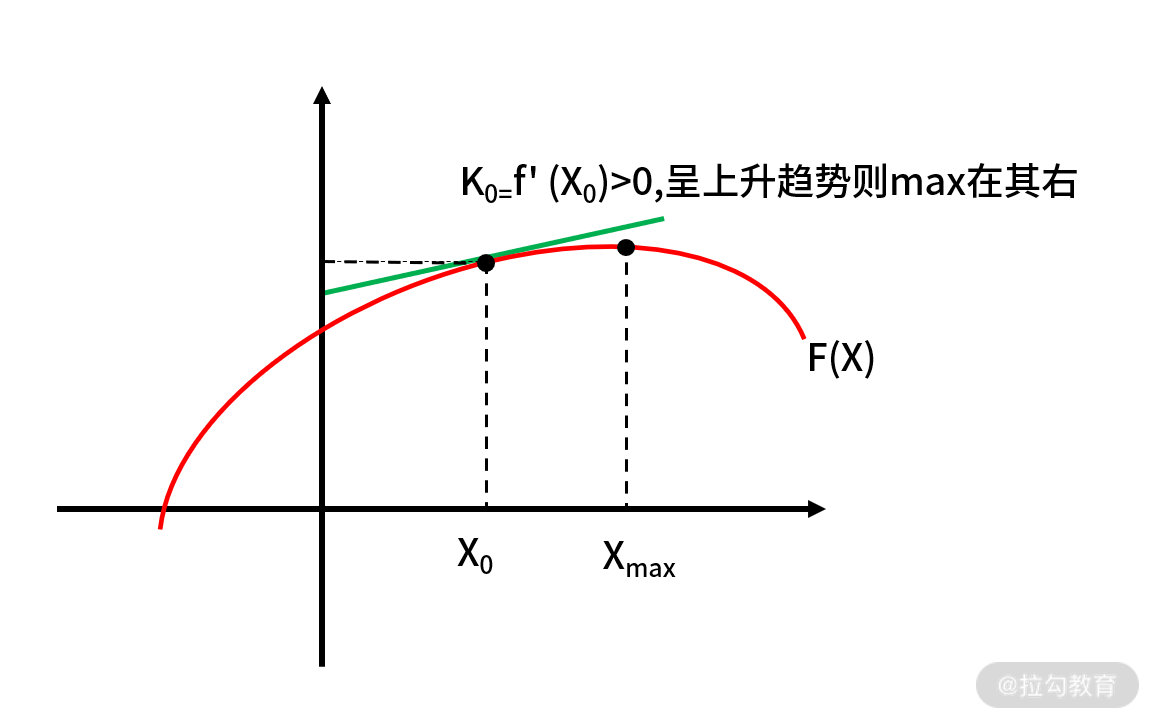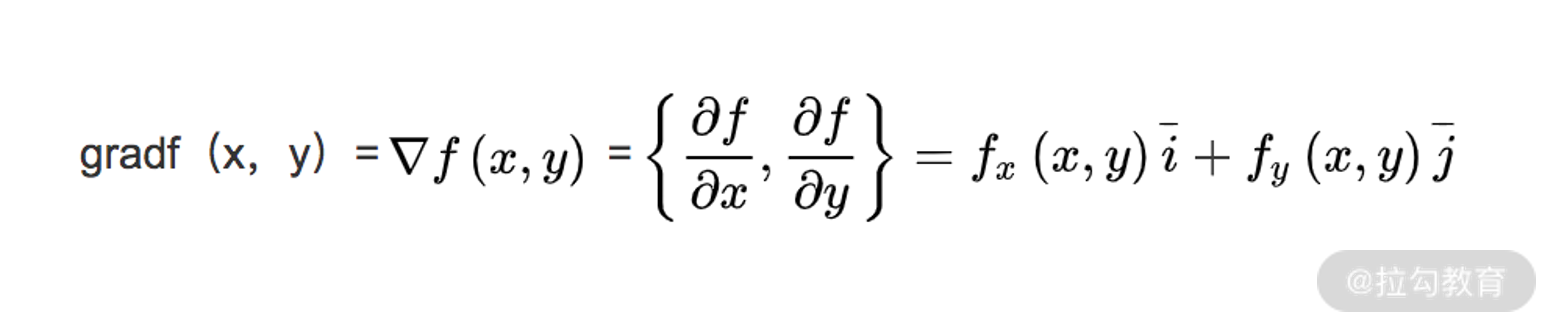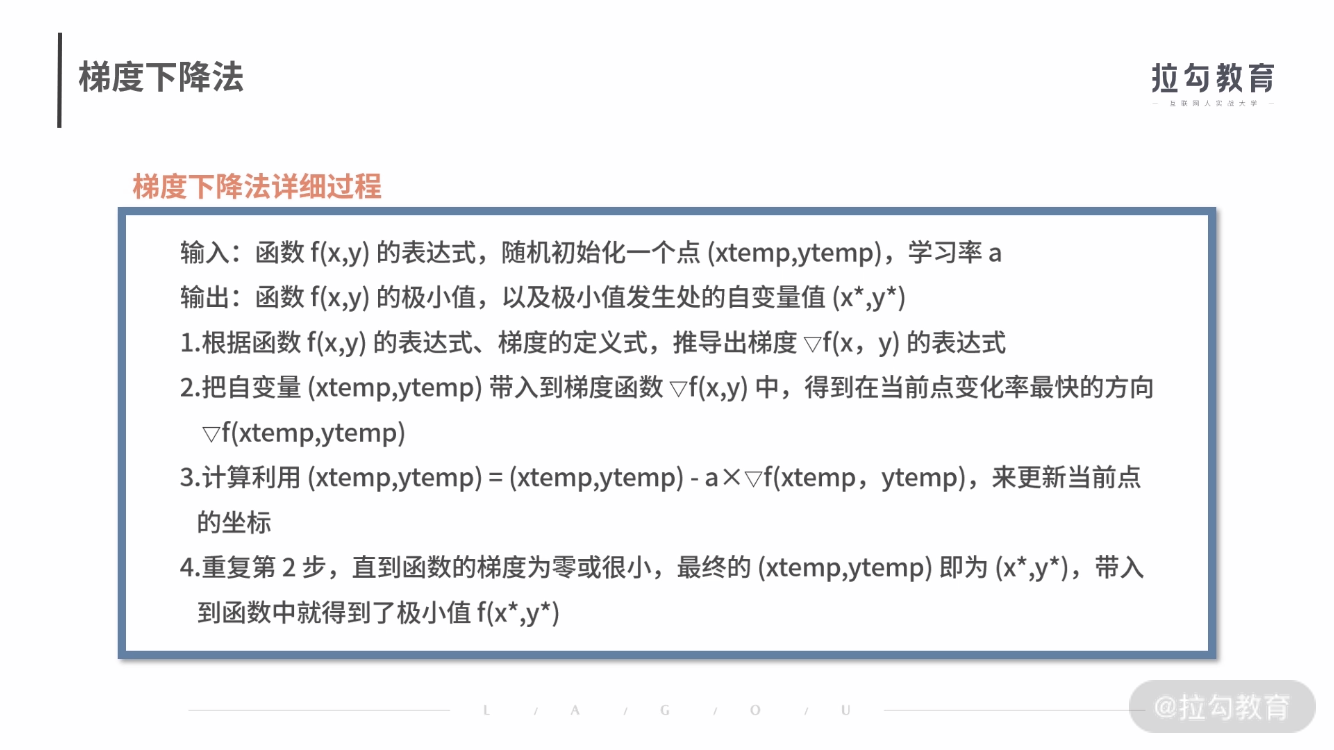• 之所以是“反方向”，是因为我们要求解的是函数的极小值；如果是极大值，就不是反方向了，公式中的“负号”就要修改为“正号”。
• “移动一小步”的实现，一般用学习率 a 来控制。通常 a 不会很大，比如设置为 0.1、0.05 等等。如果 a 过大，则可能会出现移动后“跳过”极值的可能；如果 a 过小，无非就是迭代次数多一些而已。这一步是梯度下降法最关键的步骤。

【例题2】按照之前，我们得到的商品利润函数 r 和补贴金额 x 的关系为 r(x) = p(x)×(m - x - c) = (2/(1+e-x) - 1)×(16 - x - 8)。下面利用梯度下降法，求解让利润最大的补贴额 x*。

【解析】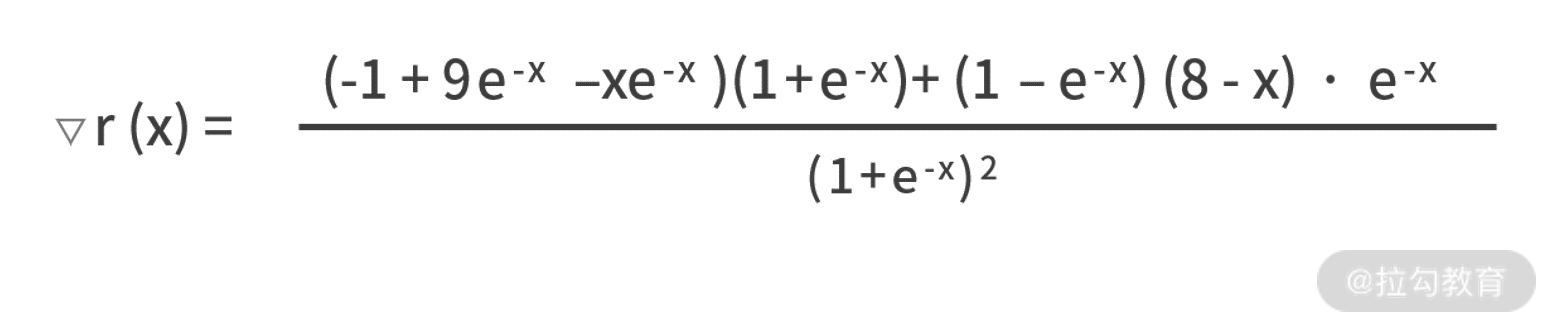import math

fenzi1 = (-1+9*math.exp(-x)-x*math.exp(-x))*(1+math.exp(-x))

fenzi2 = -(8-x)*(1-math.exp(-x))*math.exp(-x)

fenmu = math.pow(1+math.exp(-x),2)

return (fenzi1 - fenzi2) / fenmu

def main():

a = 0.01

maxloop = 1000

xtemp = 0.1

for _ in range(maxloop):

if g < 0.00005:

break

xtemp = xtemp + a*g

print xtemp

if __name__ == '__main__':

main()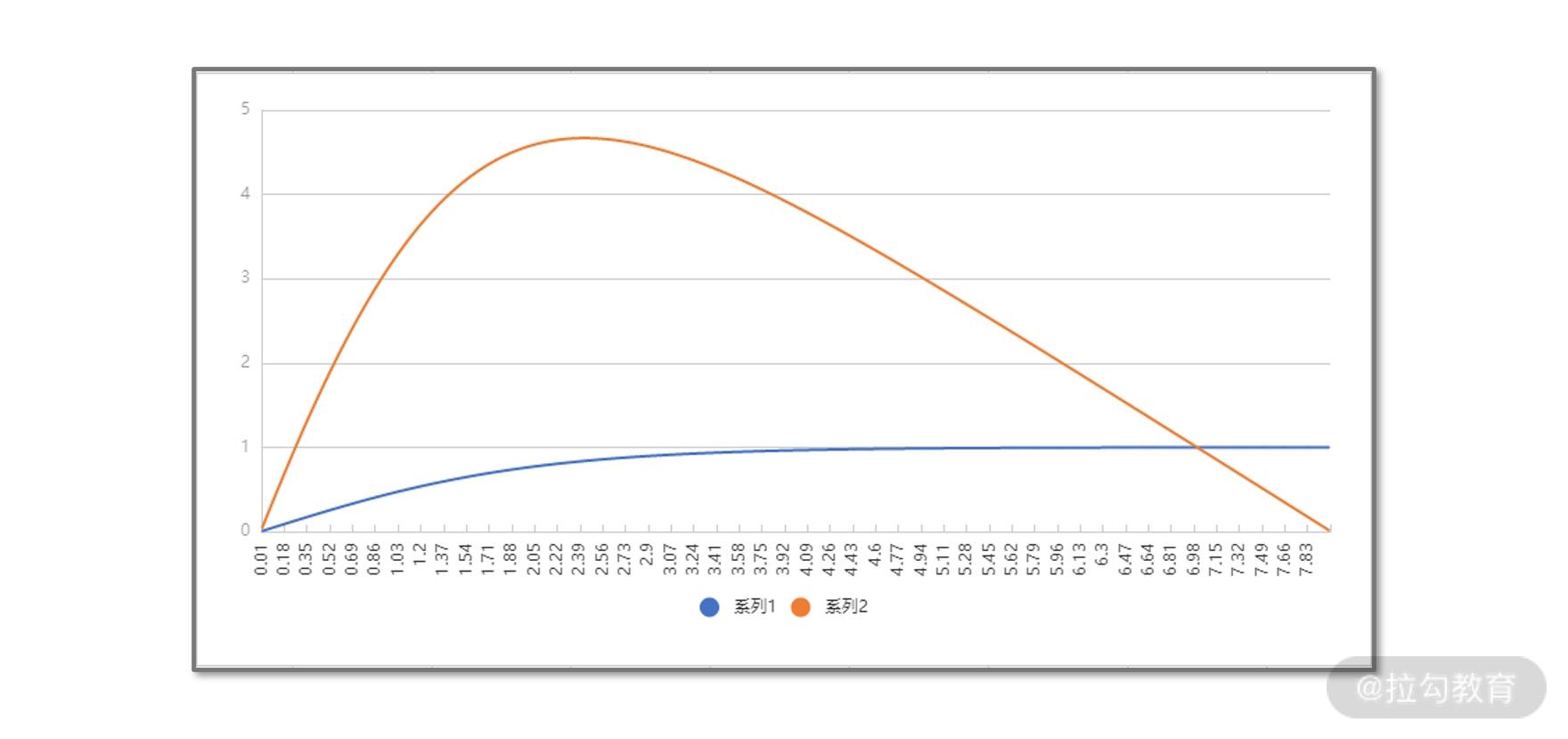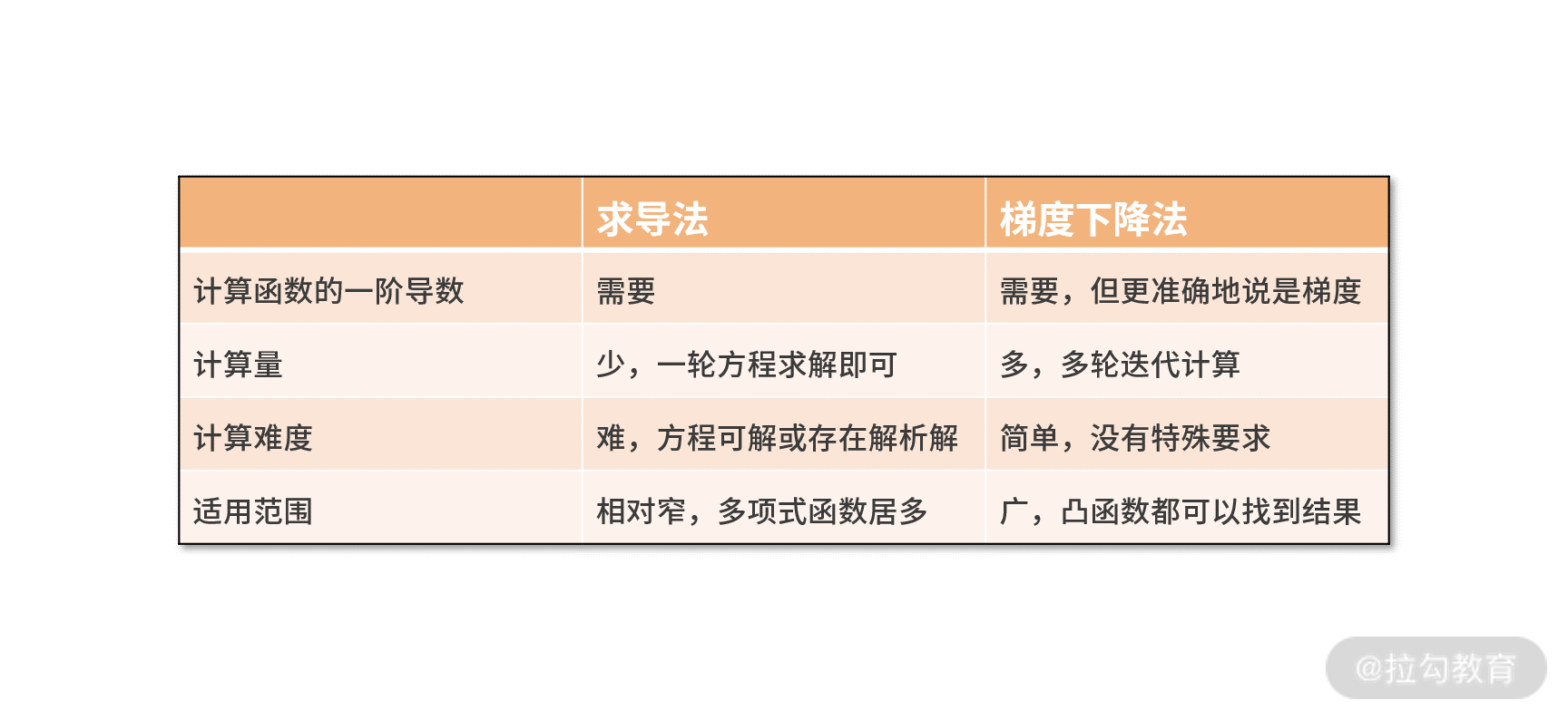• 它需要配置一些算法参数，如学习率、停止条件等。如果配置不好，可能会导致算法失效。例如，在本课时的例子中，如果学习率不小心设置为 0.7 以上，结果就不再是 2.42 了。这是因为学习率过高，导致了每一次迭代自变量“移动的步伐太大”，而频繁跨越最值无法收敛。
• 它要求目标函数为凸函数，否则就有可能会收敛到局部最优。 例如下面的双峰函数便不是凸函数。幸运的是，实际业务环境中很少会遇到非凸的函数。如果真的遇到非凸的函数，一个可行的方法是，采用随机初始化 xtemp，并多次执行梯度下降法求解。